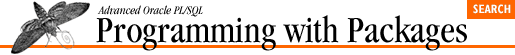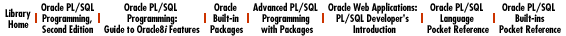home | O'Reilly's CD bookshelfs | FreeBSD | Linux | Cisco | Cisco Exam

#The load_from_dbms procedure serves as a good example of a program for loading number-text combinations from any database table into a PL/SQL table using dynamic SQL . Since you can specify the table name, WHERE clause, and column names, you can load message text from multiple sources and for multiple purposes. You can even copy this program, modify it, and use it in other programs.

The implementation of this procedure is shown in Example 9.2 . It is explained in the next section. (continued)

#### Example 9.2: The Implementation of load_ from_dbms

```PROCEDURE load_from_dbms
(table_in IN VARCHAR2,
where_clause_in IN VARCHAR2 := NULL,
code_col_in IN VARCHAR2 := 'error_code',
text_col_in IN VARCHAR2 := 'error_text')
IS
select_string PLV.max_varchar2%TYPE :=
'SELECT ' || code_col_in || ', ' || text_col_in ||
'  FROM ' || table_in;

cur INTEGER;
error_code INTEGER;
error_text VARCHAR2(2000);

PROCEDURE set_minmax (code_in IN INTEGER) IS
BEGIN
IF min_row IS NULL OR min_row > code_in
THEN
v_min_row := code_in;
END IF;

IF max_row IS NULL OR max_row < code_in
THEN
v_max_row := code_in;
END IF;
END;
BEGIN
IF where_clause_in IS NOT NULL
THEN
select_string := select_string || ' WHERE ' || where_clause_in;
END IF;

cur := PLVdyn.open_and_parse (select_string);
DBMS_SQL.DEFINE_COLUMN (cur, 1, error_code);
DBMS_SQL.DEFINE_COLUMN (cur, 2, error_text, 2000);

PLVdyn.execute (cur);
LOOP
EXIT WHEN DBMS_SQL.FETCH_ROWS (cur) = 0;
DBMS_SQL.COLUMN_VALUE (cur, 1, error_code);
DBMS_SQL.COLUMN_VALUE (cur, 2, error_text);
set_minmax (error_code);
END LOOP;
DBMS_SQL.CLOSE_CURSOR (cur);
END;```

When performing dynamic SQL , you construct the SQL statement at runtime. In load_from_dbms , I declare and initialize my SELECT string as follows:

``` select_string PLV.max_varchar2%TYPE :=
'SELECT ' || code_col_in || ', ' || text_col_in ||
'  FROM ' || table_in;```

Notice that I use all of the name arguments to the program to build the SELECT statement. There is nothing hard coded here except for the mandatory syntax elements like SELECT and FROM. That assignment (which takes place in the declaration section) covers the basic query.

What if the user provided a WHERE clause? The first line in the procedure's body adds the WHERE clause if it is not NULL:

```   IF where_clause_in IS NOT NULL
THEN
select_string := select_string || ' WHERE ' || where_clause_in;
END IF;```

Notice that you do not have to provide a WHERE keyword. That is inserted automatically by the program.

My string is ready to be parsed, so I call the PLVdyn open_and_parse procedure to take those two steps. Then I define the two columns (number and string) that are specified in the SELECT statement:

```   cur := PLVdyn.open_and_parse (select_string);
DBMS_SQL.DEFINE_COLUMN (cur, 1, error_code);
DBMS_SQL.DEFINE_COLUMN (cur, 2, error_text, 2000);```

Now the cursor is fully defined and ready to be executed. The final step in load_from_dbms is to run the equivalent of a cursor FOR loop: for every record dynamically fetched, add the text to the table and update the high and low indicators:

```   PLVdyn.execute (cur);
LOOP
EXIT WHEN DBMS_SQL.FETCH_ROWS (cur) = 0;
DBMS_SQL.COLUMN_VALUE (cur, 1, error_code);
DBMS_SQL.COLUMN_VALUE (cur, 2, error_text);```
`      set_minmax (error_code);`
```      add_text (error_code, error_text);
END LOOP;
DBMS_SQL.CLOSE_CURSOR (cur);```

I fetch a row (exiting immediately if nothing is returned). I then extract the values into local variables with COLUMN_VALUE. Following that, I update the minimum and maximum row numbers and, finally, add the text to the PL/SQL table using that same add_text program that users of PLVmsg would use to add text to the table. When I am done with the loop, I close the cursor.

To make the main body of the procedure as readable as possible, I create a local procedure ( set_minmax ) to keep track of the lowest and highest row numbers used in the PL/SQL table. This is necessary in releases of PL/SQL earlier than 2.3 since there is no way to query the PL/SQL runtime engine for this information. The local procedure, set_minmax , also serves to hide this annoying level of detail and weakness in PL/SQL table design. When you upgrade to PL/SQL Release 2.3 or above, you can just strip out this code.9.5 Integrating PLVmsg with Error Handling10. PLVprs, PLVtkn, and PLVprsps: Parsing Strings